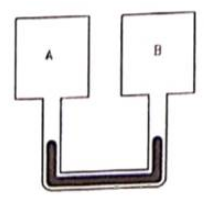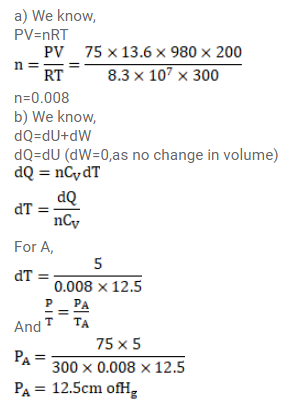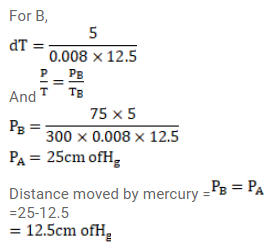# Figure shows two rigid vessels A and B each ofQuestion:

Figure shows two rigid vessels $A$ and $B$ each of volume $200 \mathrm{~cm}^{3}$ containing an ideal gas $\left(C_{\mathrm{V}}=12.5 \mathrm{~J} / \mathrm{mol}\right.$ K). The vessels are connected to a manometer tube containing mercury. The pressure in both the vessels is $75 \mathrm{~cm}$ of mercury and the temperature is $300 \mathrm{~K}$.

(a) Find the number of moles of the gas in the vessel.

(b) $5.0 \mathrm{~J}$ of heat is supplied to the gas in the vessel $A$ and $10 \mathrm{~J}$ to the gas in the vessel B. Assuming no appreciable transfer of heat from $A$ to $B$ calculate the difference in the heights of mercury in the two sides of the manometer. Gas constant $R=8.3 \mathrm{~J} / \mathrm{mol}-\mathrm{K}$.Solution: